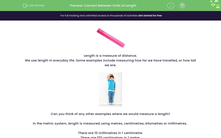# Convert Between Units of Length

In this worksheet, students will convert between units of length.Key stage:  KS 3

Curriculum topic:   Number

Curriculum subtopic:   Use Standard Units of Measure

Difficulty level:#### Worksheet OverviewLength is a measure of distance.

We use length in everyday life. Some examples include measuring how far we have travelled, or how tall we are.Can you think of any other examples where we would measure a length?

In the metric system, length is measured using metres, centimetres, kilometres or millimetres.

There are 10 millimetres in 1 centimetre.

There are 100 centimetres in 1 metre.

There are 1,000 metres in 1 kilometre.Look at this diagram that you can use to help you convert between the various units:If we have a quantity measured in centimetres and we want to change it to millimetres how do do we do this?

We want the arrow pointing from cm to mm, so we multiply by 10.

Example:

Change 15 cm to mm

15 x 10 = 150

So 15 cm = 150 mm

And if we want to change a quantity from metres to kilometres? We're going the opposite way, so we divide by 1,000.

Example:

Change 7,531 metres into kilometres:

7,531 ÷ 1,000 = 7.531

So 7,531 metres = 7.531 kilometres